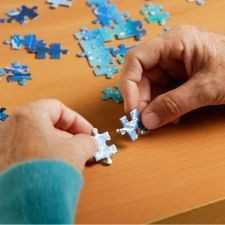Brainy Puzzles
Puzzle Solvers: Interesting brainy puzzles shared by fellow puzzlers
215 Members
Join this group to post and comment.puzzle for you

hi.... try this puzzle

there are two solutions containing water & milk in the ratio 1:4 & 1:5.
now they mixed. so what is the ratio of water & milk in new solution
the given choices..
a) 2:9 b)5:9 c)9:20 d)5:20rick • Aug 9, 2006
hi.... try this puzzle

there are two solutions containing water & milk in the ratio 1:4 & 1:5.
now they mixed. so what is the ratio of water & milk in new solution
the given choices..
a) 2:9 b)5:9 c)9:20 d)5:20
Let there be 30 litres of each solution.

So quantity of Water & Milk in first solution is 6 litre & 24 litre, respectively.

similarly, quantity of Water & Milk in second solution is 5 litre & 25 litre, respectively.

If the two solutions are added, total quantity of solutions = 60 litre.
Qutantity of water = 6 + 5 = 11 litre.
Quantity of milk = 24 + 25 = 49 litre.

Required ratio = 11/49

😒 ... have you verified the options?

Ricki tried and got the same ans as yours but it is not in the option..😕rick • Aug 9, 2006
Don't worry, the options are wrong 😁 . The approach is right and all the steps are right. The answer has to be right !

Rickkarthick200621 • Aug 9, 2006
result

water : 1/5 + 1/6
milk : 4/5 + 5/6

no option there

always try to do it simple and save timeaashima • Aug 9, 2006
well

i too got the same answer as 11:49 😔 aditi i suppose there lies some fault in the question only then.Neha • Aug 10, 2006
Same here,even I got the answer as 11:49..check the options again.neha
Same here,even I got the answer as 11:49..check the options again.

I am pretty sure that there is no crap in this question...

Only Choice's are wrong...
Just explainnig a bit why rick take 30 liters in his assumption...
in first solution ratio is 1:4 so (total 5 say..) in second solution ratio is 1:5 (total 6 say..) and to reduce the fractions calculation he tool the LCM of 5 and 6 so it will reduce his calculations...Kaustubh Katdare • Sep 23, 2006
((bumpp))

-The Big K-rick
Let there be 30 litres of each solution.

So quantity of Water & Milk in first solution is 6 litre & 24 litre, respectively.

similarly, quantity of Water & Milk in second solution is 5 litre & 25 litre, respectively.

If the two solutions are added, total quantity of solutions = 60 litre.
Qutantity of water = 6 + 5 = 11 litre.
Quantity of milk = 24 + 25 = 49 litre.

Required ratio = 11/49

😒 ... have you verified the options?

Rick
I don't know how i missed this one but I think rick u are exactly on the right track but the point where my solution differs is if you are considering 1part of water as 6 for the first solution then why are you taking the same unit i.e. 1 unit of same liquid i.e. water as 5 in the other solution? So worked it out that way it will come to 2:9 which exists in the options provided. so aditi whats the answer? i think its long time plz post in the answer.😁Jerry • Sep 25, 2006
integratdbrains
I don't know how i missed this one but I think rick u are exactly on the right track but the point where my solution differs is if you are considering 1part of water as 6 for the first solution then why are you taking the same unit i.e. 1 unit of same liquid i.e. water as 5 in the other solution? So worked it out that way it will come to 2:9 which exists in the options provided. so aditi whats the answer? i think its long time plz post in the answer.😁
I could not get your point. I think Rick has the perfect answer. How did you get 2:9 ?

JerryActually jerry i donot claim to be correct so lets wait for aditi to post the answer then if its correct i will surely post in the solution! 😀Mayur Pathak • Sep 26, 2006

I think explaination by integratd brains is right.hi all......
i dont know the answer.. i found it somewhere and thought to put it on ce...Kaustubh Katdare • Sep 27, 2006
Math Guru drops in

(bump) (bump)

Math Guru drops in 😎 ! I noticed that we have conflicting solutions for a stupid math problem and decided to separate milk & water.

The trouble starts here -

Let there be 30 litres of each solution.
Does the problem mention that we have "equal quantities" of both the solutions? Why assume?

The problem is as simple as
"1 part of milk from first solution mixes with 1 part of milk from second solution"

&

"4 parts of water mix with 5 parts of water from second solution"

Therefore, the new solution will have Milk:Water :: 2:9 😁

I hope this helps. Remember: Keep It Simple & Stupid!

-The Big K-Mahesh • Oct 7, 2006
Hi Biggie,
Can u explain same soln if we take equal quantities that to same 30L?Kaustubh Katdare • Oct 7, 2006
Mahesh
Hi Biggie,
Can u explain same soln if we take equal quantities that to same 30L?
Mahesh, the problem does not talk about equal quantities. The reasoning by Rick is perfect except that we cannot have equal quantities of the solutions.

-The Big K-gautyaaa • Feb 13, 2007

in 1st sol.
water----->1lt
milk---->4lt
in 2nd sol.
water---->1lt
milk---->5lt
total---->11lt.

check in d options ---->2+9==11😎

how can u question d question....lol😁